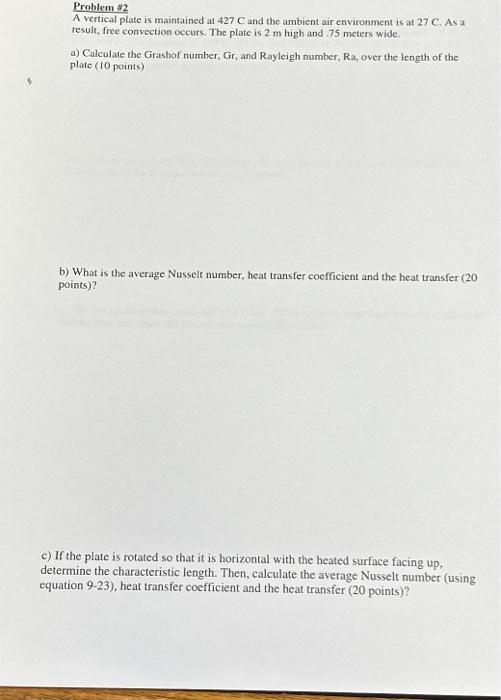# (Solved): please be neat and show all steps. Problem \#2 A vertical plate is maintained at 427C and the ambien ...

please be neat and show all steps.Problem \#2 A vertical plate is maintained at and the ambient air environment is at . As a result, free convection occurs. The plate is high and 75 meters wide. a) Calculate the Grashof number, Gr, and Rayleigh number, Ra, over the length of the plate ( 10 points) b) What is the average Nusselt number, heat transfer coefficient and the heat transfer ( 20 points)? c) If the plate is rotated so that it is horizontal with the heated surface facing up, determine the characteristic length. Then, calculate the average Nusselt number (using equation 9-23), heat transfer coefficient and the heat transfer ( 20 points)?

We have an Answer from Expert